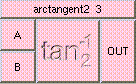ARCTANGENT2THE BOX'S MAIN HELP: This function returns a scalar between [-PI,PI]. The sign of the inputs determines which quadrant the result will be. Arctangent is the inverse of the tangent function. atan2(b,a) = y/x if and only if tan(y/x) = b/a. A (input): This may be any real number. The sign of the inputs determines which quadrant the result will be. B (input): This may be any real number. This is the longitudinal angle. The sign of the inputs determines which quadrant the result will be. OUT (output): This function returns a float between [-PI,PI]. The sign of the inputs determines which quadrant the result will be. Arctangent is the inverse of the tangent function. atan2(b,a) = y/x if and only if tan(y/x) = b/a.

<-- BACK TO Math